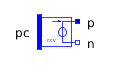Add - MapleSim Help

Output the sum of the two inputsDescription The Complex Add (or Add) component computes the scaled sum of the two complex inputs ${u}_{1}$ and ${u}_{2}$. To use the complex conjugate of ${u}_{1}$ or ${u}_{2}$, set Use Conjugate Input 1 or Use Conjugate Input 2 to $\mathrm{true}$, respectively. A complex parameter value may be entered using the complex constructor, for example, Complex(3,4), or using I for the imaginary unit, 3+4*I. If a purely real value is entered, the real value will be converted to its complex equivalent.Equations $y={k}_{1}{u}_{1}+{k}_{2}{u}_{2}$Connections

 Name Description Modelica ID ${u}_{1}$ Complex input signal 1 u1 ${u}_{2}$ Complex input signal 2 u2 $y$ Complex output signal yParameters

 Name Default Units Description Modelica ID Use Conjugate Input 1 $\mathrm{false}$ If true, use the complex conjugate of input 1 useConjugateInput1 Use Conjugate Input 2 $\mathrm{false}$ If true, use the complex conjugate of input 2 useConjugateInput2 ${k}_{1}$ $1$ Gain of upper input k1 ${k}_{2}$ $1$ Gain of lower input k2Modelica Standard Library The component described in this topic is from the Modelica Standard Library. To view the original documentation, which includes author and copyright information, click here.